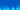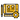# How to Sort a List by Proximity of Location in Sencha Touch

Originally published June 11, 2014

We can use GPS and other Geolocation methods to create cool location based functionality in our mobile applications. One thing you will probably have seen a lot of are apps that have 'Near You' lists – these might be things like shops, people, events and so on that are sorted by how close the user is to the point of interest.

On the surface, this seems like something that would be pretty complex – but thanks to mathematicians of the past and other smart people who have already come up with formulas for calculating these things it's not too difficult to implement in a Sencha Touch application.

### How do we sort a Sencha Touch list normally?

Let's take a step back first and cover how to sort a list in Sencha Touch by something a lot simpler, let's say alphabetical order.

In Sencha Touch a list pulls in its data from a Store, and we can control where items are displayed in a list by sorting the store. If we have a store called 'Robots' and we wanted to sort the robots alphabetically by their name we would do something like this:

``````var robotStore = Ext.getStore('Robots');
robotStore.sort('name', 'ASC');``````

and if we wanted reverse alphabetical order:

``robotStore.sort('name', 'DESC');``

### Let's throw latitude and longitude into the mix

Sorting by distance based on comparing the latitude and longitude of the users current location and that of points around them is a little more difficult. We can't sort by latitude and longitude by themselves, but we can use them to calculate the distance between two points and then sort by that.

We'll start by grabbing the users current location using the following code:

``````var options = { enableHighAccuracy: true };
navigator.geolocation.getCurrentPosition(
MyApp.util.Geolocation.onSuccess,
MyApp.util.Geolocation.onError,
options
);``````

You will notice this code contains two callback functions 'onSuccess' and 'onError' which go to a utility file called 'Geolocation'. If you don't already have a util folder, create one at MyApp/app/util and then create a file called 'Geolocation.js' in that folder. We're creating this utility file to help us out with some of the geolocation functions.

Once you've created that file, place the following code inside:

``````Ext.define('MyApp.util.Geolocation', {
singleton: true,

onSuccess: function (position) {
var store = Ext.getStore('SomeStore');
callback: function (records, operation, success) {
store.each(function (location) {
var lat2 = parseFloat(location.get('lat')) || 0;
var lon2 = parseFloat(location.get('lng')) || 0;
if (lat2 && lon2) {
var distance = playgrounds.util.Geolocation.getDistance(
position.coords.latitude,
position.coords.longitude,
lat2,
lon2
);
location.set('distance', distance);
}
}, this);
store.sort('distance', 'ASC');
},
});
},

getDistance: function (lat1, lon1, lat2, lon2) {
var R = 6371;
var dLat = this.deg2rad(lat2 - lat1);
var dLon = this.deg2rad(lon2 - lon1);

var a =
Math.sin(dLat / 2) * Math.sin(dLat / 2) +
Math.sin(dLon / 2) * Math.sin(dLon / 2) * Math.cos(lat1) * Math.cos(lat2);
var c = 2 * Math.atan2(Math.sqrt(a), Math.sqrt(1 - a));
var d = R * c;
return d;
},

onError: function (error) {
},

``store.sort('distance', 'ASC');``